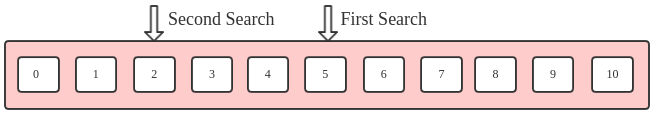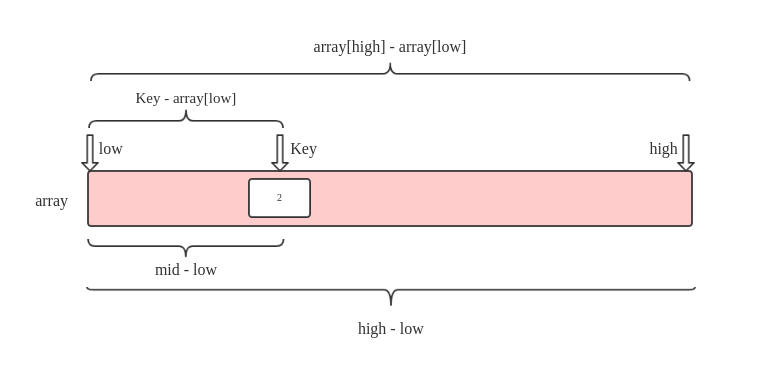># Binary Search

Binary Search is a basic search algorithm. Its basic idea is each search is reduced by half of the data. Such as，using Binary Search to search 2 in this table :1. First  search index = (0 + 10) / 2 = 5，and 2 < 5，then search [0, 4]
2. Second search index = (0 + 4)  / 2 = 2, and 2 == 2，find it !


Before you use Binary Search，you must be know below points :

1. Binary Search O(n) = O(log2n)
2. The set of searchs it must be ordered（Ascending or Descneding），such as above array table.
3. It applies to infrequently changing and finding frequent ordered lists.


Now，we see the algorithm code.

## Algorithm Code

I use C to coding，like below :

### Array and division

Using arrays and division is the easiest way :

int *binary_search_common(int *array, int length, int key) {
int low = 0;
int mid = 0;
int high = length - 1;

while (low <= high) {
mid = (high + low) / 2;
printf("binary_search_common: mid = %d\n", mid);

if (key < array[mid])
high = mid - 1;
else if (key > array[mid])
low = mid + 1;
else
return array + mid;	// Ok，find it !
}

// No find !
return NULL;
}


### Point and right shift

Using pointer and right shift instead of array and division to improve efficiency :

int *binary_search_improve(int *array, int length, int key) {
int *low = array;
int *mid = NULL;
int *high = array + length - 1;

while (low <= high) {
mid = low + ((high - low) >> 1);
printf("binary_search_improve: mid = %d\n", mid - low);

if (key < *mid)
high = mid - 1;
else if (key > *mid)
low = mid + 1;
else
return mid;	// Ok，find it !
}

// No find !
return NULL;
}


### Recursive

I suggest youd better use recursion to achieve it again :

int *binary_search_rec(int *array, int *low, int *high, int key) {
if (low > high)
return NULL;	// No find !

int *mid = low + ((high - low) >> 1);
printf("binary_search_rec: mid = %d\n", mid - low);

if (key < *mid)
return binary_search_rec(array, low, mid - 1, key);
else if (key > *mid)
return binary_search_rec(array, mid + 1, high, key);
else
return mid;	// Ok，find it !
}


### TestCode

I use an order array as test data :

int main(int argc, char **argv) {
if (argc < 2) {
printf("Usage: ./a.out search_num:[0, 10]\n");
exit(1);
}

int a = { 0, 1, 2, 3, 4, 5, 6, 7, 8, 9, 10 };
int key = atoi(argv);

int *find1 = binary_search_common(a, 11, key);
int *find2 = binary_search_improve(a, 11, key);
int *find3 = binary_search_rec(a, a, a + 11, key);

printf("%d\n", *find1);
printf("%d\n", *find2);
printf("%d\n", *find3);
return 0;
}


Compile and run :

gcc binary_search.c
./a.out 2

binary_search_common: mid = 5
binary_search_common: mid = 2
binary_search_improve: mid = 5
binary_search_improve: mid = 2
binary_search_rec: mid = 5
binary_search_rec: mid = 2
2
2
2


Can be seen，we only need to find 2 times. But，for an ordered list，it is not the fastest algorithm. For example，finding 2 still takes 2 times，but actually we can find 2 only once !

Continue to see the faster algorithm below !

Interpolation Search and Binary Search basically the same，the only difference is how to calculate mid.

In Binary Search :

mid = low + ((high - low) >> 1);


But in Interpolation Search :

mid = low + (high - low) * ((double)(key - array[low]) / (array[high] - array[low]));


What is it ? look below :We calculate the mid by the following proportional formula :

(mid - low) / (high - low) = (key - array[low]) / (array[high] - array[low])


->

mid = low + (high - low) * (key - array[low]) / (array[high] - array[low]);


But we must be add double to case type，because we need to let 0 < (key - array[low]) / (array[high] - array[low]) < 1if we do not case it to double，the expression will be zeor，so we must be case it to double :

// Case (key - array[low]) to double.
mid = low + (high - low) * ((double)(key - array[low]) / (array[high] - array[low]));


Point type，because point do not add to double，we need to case it to int so that it can add to point low :

mid = low + (int)((high - low) * ((double)(key - (*low)) / ((*high) - (*low))));


### Interpolation Code

OK，now you only need to replace how to calculate the expression of mid can be achieved Interpolation Search :

int *binary_search_xxx(int *array, int length, int key) {
...
while (low <= high) {
// For array and division.
mid = low + (high - low) * ((double)(key - array[low]) / (array[high] - array[low]));
printf("binary_search_xxx: mid = %d\n", mid);

// For point and >>
mid = low + (int)((high - low) * ((double)(key - (*low)) / ((*high) - (*low))));
printf("binary_search_xxx: mid = %d\n", mid - low);
}
...
}


You can use the above code main function to test this algorithm，and the result like below :

gcc binary_search.c
./a.out 2

binary_search_common: mid = 2	// Note: We only need to search once !
binary_search_improve: mid = 2
binary_search_rec: mid = 2
2
2
2
`

This can be，thank you for reading.

DLonng at 06/13/17